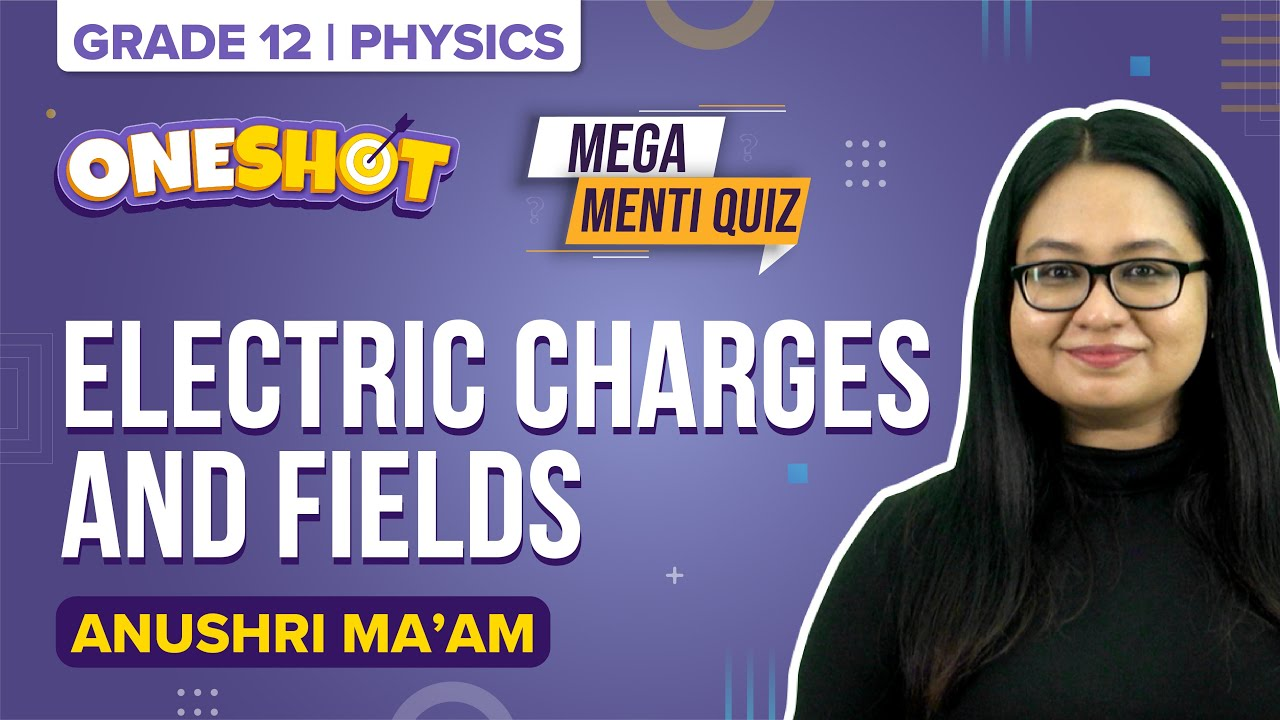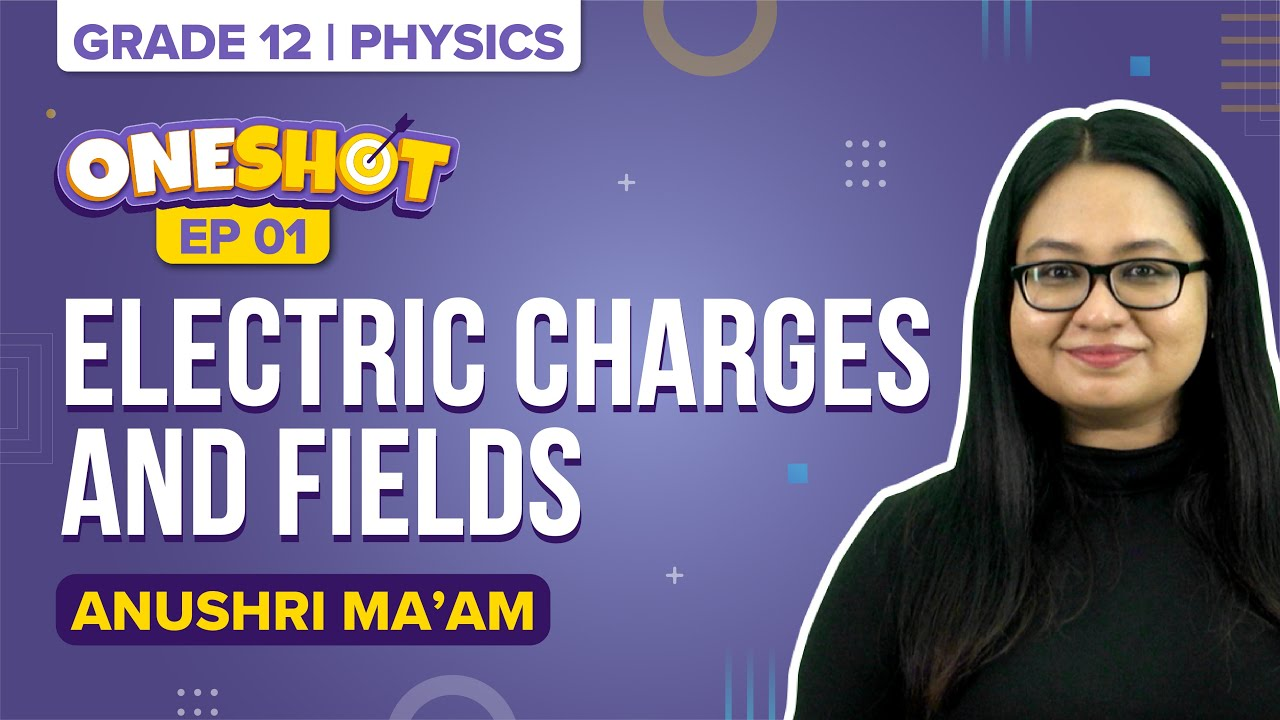# Electric Charges And Fields MCQs for NEET

A charge is a property associated with the matter due to which it experiences and produces an electric and magnetic field.

• Charges are scalar in nature and they add up like real numbers. (Additivity of Charges)
• The total charge of an isolated system is always conserved. ( Conservation of Charge)
• Charges exist in a discrete amount rather than continuous value. Hence, quantised. (Quantisation of Charge)

1. Pick the correct statement

1. The glass rod gives protons to silk when they are rubbed against each other.
2. The glass rod gives electrons to silk when they are rubbed against each other.
3. The glass rod gains protons from silk when they are rubbed against each other.
4. The glass rod gains electrons when they are rubbed against each other.

Answer: (b) The glass rod gives electrons to silk when they are rubbed against each other.

2. A force F acts between two charges +Q and -Q that is placed at a certain distance from each other. The third sphere of charge Q is placed between them. What is the magnitude and force experienced by the third charge?

1. 3F in the direction of +Q charge
2. 4F in the direction of +Q charge
3. 8F in the direction of -Q charge
4. No direction and magnitude is zero

Answer: (c) 8F towards -Q charge

3. At the four corners of the square A, B, C and D, Four charges of equal magnitude q are placed. Determine the magnitude of the force acting on the charge at point B?

1. $$\begin{array}{l}\frac{(\frac{2+1}{\sqrt{2}})q^2}{4\pi \varepsilon _0a^2}\end{array}$$
2. $$\begin{array}{l}\frac{(1+2\sqrt{2})q^2}{2\times 4\pi \epsilon _0a^2}\end{array}$$
3. $$\begin{array}{l}\frac{4q^2}{4\pi \epsilon _0a^2}\end{array}$$
4. $$\begin{array}{l}\frac{3q^2}{4\pi \epsilon _0a^2}\end{array}$$

$$\begin{array}{l}\frac{(1+2\sqrt{2})q^2}{2\times 4\pi \epsilon _0a^2}\end{array}$$

4. At a distance r, two equal charges are kept and they exert a force F on each other. What is the force acting on each charge, if the distance between them is doubled and charges are halved?

1. F/4
2. 4 F
3. F/16
4. F/8

5. Determine the total electric flux of a unit positive charge which is kept in the air?

1. $$\begin{array}{l}{\epsilon _0}^{-1}\end{array}$$
2. $$\begin{array}{l}{\epsilon _0}\end{array}$$
3. $$\begin{array}{l}4\pi \epsilon _0\end{array}$$
4. $$\begin{array}{l}{4\pi \epsilon _0}^{-1}\end{array}$$

$$\begin{array}{l}{4\pi \epsilon _0}^{-1}\end{array}$$

6. The variation of electric field E due to a hollow spherical conductor of radius R as a function of distance from the centre of the sphere is shown in which of the following graphs?

1.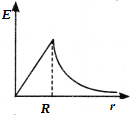2.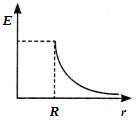3.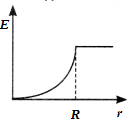4.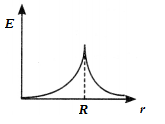Answer: (b)7. Pick the correct statement from the following

1. If the point has a charge then the electric field is discontinuous at the point.
2. Continous electric field at a point
3. Continous electric field at a point if a charge is present at the point.
4. At the point, the electric field is discontinuous if a negative charge is present at the point.

Answer: (a) If the point has a charge then the electric field is discontinuous at the point.

8. When is Gauss law true?

1. the speed of light isn’t a universal constant
2. the inverse square law is false
3. magnetic monopoles are present
4. None of the above

Answer: (b) the inverse square law is false

9. What is the SI unit of permittivity of free space?

1. Weber
3. C2N-1m-2
4. C2N-1m2

10. Pick the true statements about electric field lines

1. It provides information about the direction of the electric field
2. Electric field lines provide information about the type of charge
3. Electric field lines provide information about the field strength
4. All of the above

Answer: (d) All of the above

11. The electric field lines due to a single negative charge are represented by

1.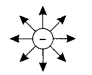2.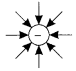3.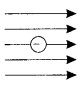4.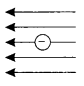12. Which surface is considered for Gauss law?

1. Spherical surface
2. Closed Surface
3. Gaussian Surface
4. Plane Surface